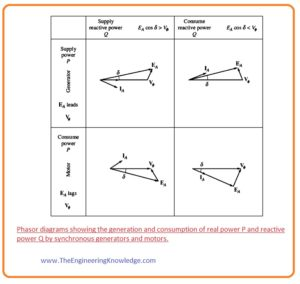Hello, readers welcome to the new post. In this psot we will discuss Difference between Synchronous Motor & Synchronous Generator. The motor is a device that is used to transform electrical energy into mechanical energy and generator is used to transform mechanical energy into electrical energy.

In this post, we will learn some basic differences between Synchronous Motor & Synchronous Generator and finds their related parameters. So let get started.

What is Synchronous Motor

• The Synchronous Motor is such a motor in which speed of rotation of the rotor is identical to the synchronous speed, synchronous speed is rotational speed of the magnetic field provided at the stator of the motor.
• The stator of the motor is electromagnetic that produced field when current is provided at stator that rotating speed of the field is called synchronous speed.
• Synchronous motor is also known as the doubly feed because its rotor and stator both are connected with the spate inputs supply.
• Generally used alternating current motors are induction and synchronous motors.
• The main difference between these 2 motors is that synchronous motor rotates on a constant speed that is similar to the stator’s rotating field speed.
• The rotor of a synchronous motor behaves like permanent magnetic or electromagnetic and its field interacts with the stator field than it rotates at a time with the rotation of the stator field.
• While in induction motor slip is required, that means the speed of the rotation of the rotor is less than the speed of the rotating field at stator.What is Synchronous Generator

• The synchronous generator is also known as an alternator, it converts the mechanical power into the electrical.
• The electric energy we used in our home or industries is mostly produced by the synchronous generator.
• There are many sources of energy conversion in the world but most of the energy is converted by the synchronous generator

#### Working Principle of Synchronous Generator

• The working of a synchronous generator is based on Faraday’s Law of electromagnetic induction.

emf= dΦ/dt

• This law says that the rate of change of flux in any device will produce emf in that device. If a device is static and the field is rotating it will also produce field in the device.
• In case of a synchronous generator, the rotor is rotating, and it produces field in the stator.
• For an understanding of emf induced in any device study the article on the voltage induced in the loop
• All these 4 possibilities of these machines are shown in a given figure in the shape of the phasor diagram.

###Equivalent Circuit of a Synchronous Motor

• Almost all aspects of synchronous motor like construction, working, is similar to the synchronous generator but the difference is that generator converts mechanical power into electrical and motor converts electrical into mechanical.
Due to this power flow direction will be against the generator’s power flow and current will also reverse.
• So the equivalent circuitry of the synchronous motor will be similar to synchronous generator equivalent circuit but the current IA flow direction will be opposite to generator.
• The resultant circuit of a synchronous motor is shown in a given figure.• The per-phase equivalent circuitry of motor is drawn in this figure.• If we apply KVL (Kirchhoff’s voltage law) on an equivalent circuit of the synchronous motor then we have these equations.
Vø= EA + jXSIA + RAIA

EA = Vø – jXS IA – RAIA

These equations are similar to the synchronous generators but the difference is that sign is different

# Free SMT Assembly Monthly

New arrival for Aluminum boards, only \$2

## New users enjoy \$54 free coupons after signing up at JLCPCB successfully

\$54 coupons can also apply to 3D printing orders, for 3D printing special offer, it gets started at \$1